##### ASVAB AFQT For Dummies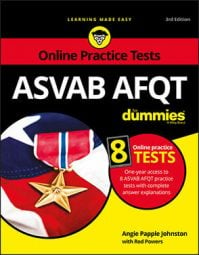Two of the four subtests that make up your AFQT score are math tests. The first, Arithmetic Reasoning, tests your ability to use mathematics to solve various problems that you may encounter in real life — in other words, math word problems. The second, Mathematics Knowledge, tests your ability to solve general math problems. Here are some tips to help maximize your ASVAB math subtest scores:
• Memorize the math order of operations. Start by working everything in parentheses. Then move on to exponents. Then perform any multiplication and division. End with addition and subtraction. An easy way to remember this order is to think of the phrase, “Please Excuse My Dear Aunt Sally” (Parentheses, Exponents, Multiply, Divide, Add, Subtract). While this order is flexible in some situations, knowing the basic order will help you maximize your score.

• Remember the apples-and-oranges rule. Be sure to convert units of measurement so that they’re consistent. If a question asks how many 3-x-6-inch bricks it will take to cover a 6-x-6-foot patio, be sure to recognize that inches and feet are two different measurements and perform the necessary conversions.

• Memorize common math formulas before the day of the test. These formulas are not provided for you at the testing center. After you receive your scratch paper, take a moment before the test starts and write down all the formulas you’ve memorized. Here’s a list of some of the formulas you need to know:

• Perimeter of a square: p = 4s, where s = one side of the square

• Area of a square: a = s2

• Diagonal of a square: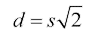• Perimeter of a rectangle: p = 2l + 2w, where l = the length and w = the width of the rectangle

• Area of a rectangle: a = lw

• Diagonal of a rectangle: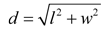, where d = the diagonal, l = the length, and w = the width of the rectangle. This formula is the Pythagorean theorem solved for the hypotenuse (c) — it just uses different letters.
• Perimeter of a triangle: p = s1 + s2 + s3, where s = the length of each leg of the triangle

• Area of a triangle: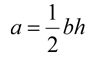, where b = the length of the triangle’s base (bottom) and h = the height of the triangle
• Pythagorean theorem: a2 + b2 = c2, where c equals the length of a right triangle’s hypotenuse, and a and b equal the lengths of the remaining two legs of the right triangle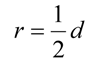, where d = the diameter of the circle
• Diameter of a circle: d = 2r

• Circumference of a circle: c = 2Πr

• Area of a circle: a = Πr2

• Volume of a cube: v = s3, where s = the length of one side of the cube

• Volume of a rectangular box: v = lwh, where l = the length, w = the width, and h = the height of the box

• Volume of a cylinder: v = Πr2h, where r = the radius of the cylinder and h = the height of the cylinder

• Surface area of a cube: SA = 6s2

• Surface area of a rectangular box: SA =2lw + 2wh + 2lh

• Use the scratch paper provided to help you solve problems. Draw a picture for math word problems to help you visualize the situation and pick out the relevant information. Remember: You can’t use a calculator on any of the AFQT subtests.

• For difficult problems, try plugging the possible answer choices into the equation to see which one is right.

• Learn to separate the meat from the fat, and select only the information needed to solve the problem. Math word problems often provide extra information to confuse you.